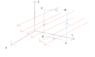# Can a magnetic field ever cause a translation motion?

Lorentz Law says that for a charged particle moving with a velocity v in a magnetic field B then the force on it is given by $$\mathbf{F} = q (\mathbf{v} \times \mathbf{B})$$
Now, if I say that particle’s velocity and the magnetic field are aligned then according to Lorentz Law there will be no force on it and hence no attraction.
If we have something that have a magnetic moment $\mathbf{\mu}$ then the torque produced by magnetic field on it is $$\tau = \mathbf{\mu} \times \mathbf{B}$$
So, magnetic field seems to me to work only in rotational aspects and never causes any translational effect. I want to know if this is true.

•Delta2

Mentor
There can be a translational force on a magnetic moment given by ##\vec F=\nabla(\vec \mu \cdot \vec B)##

•Staff Emeritus
Can a magnetic field ever cause a translation motion?

What happens when a piece of iron gets near a magnet?

•gmax137
Homework Helper
Gold Member
I wonder where this thread will end up, thinking it might go towards the "is the magnetic field capable of doing work on matter?" way...

•There can be a translational force on a magnetic moment given by ##\vec F=\nabla(\vec \mu \cdot \vec B)##
But why it is taught that magnetic dipole rotates in a field and never mentions that after being aligned it will move? I mean a side of dipole going to feel a force just equal and opposite to the other side, hence translational effect is not caused.

I wonder where this thread will end up, thinking it might go towards the "is the magnetic field capable of doing work on matter?" way...
I quite didn’t get you.

What happens when a piece of iron gets near a magnet?
That was the real problem and because of that I asked this question.

Homework Helper
Gold Member
With a completely uniform magnetic field in a given direction, there are no forces along the direction of the field. However, from a magnetic pole, you always get some spreading of the field lines, which means besides a ## B_z ##, you get some finite ## B_x ## and/or ## B_y ##. This same question came up a year or two ago, but I don't have that post at my fingertips. I'll try doing a "search". Yes, I found it: See https://www.physicsforums.com/threa...elds-and-magnetic-moment.875780/#post-5500177

Last edited:
•Mentor
But why it is taught that magnetic dipole rotates in a field and never mentions that after being aligned it will move? I mean a side of dipole going to feel a force just equal and opposite to the other side, hence translational effect is not caused.
My class mentioned it. There is no translational force in a uniform B field. Perhaps your professor only got to cover uniform fields and ran out of time to cover non uniform fields in that lecture. You would have to ask the teacher why they didn’t cover it.

By the way, the magnetic moment doesn’t need to be aligned to have a force. It just can’t be perpendicular.

•berkeman
I think I should express myself more clearly. Consider this image in attachment, in the image the red arrows represent the magnetic field B which is given by $\mathbf{B} = B_0 \hat i$ and in the blue square loop a current I is flowing and it's direction is mentioned by the arrows in each arm. The magnetic moment $\mathbf{\mu}$ is aligned with the field.
Now, by Laplace's equation force on each arm is $$\mathbf{F} = I\mathcal{l} \times \mathbf{B}$$
$$\mathbf{F_{AB}} = I (\mathbf{AB} \times \mathbf{B})$$
$$\mathbf{F_{AB}} = I~a~B_0 \hat j$$ we can assume that our loop is a square with all sides equal to a . Similarly, force on the arm CD is $$\mathbf{F_{CD}} = - I~a~B_0 \hat j$$
$$\mathbf{F_{AB}} + \mathbf{F_{CD}} = 0$$ which shows that there is no translational effect as the net force is force (we can do the similar calculations for the arms BC and AD) and the result would that $\mathbf{F_{net}} = 0$ which is the condition for translational equilibrium.
I request you all to please explain me through an analysis like this.

#### Attachments

•Screen Shot 2019-11-28 at 4.23.02 PM.png
12.8 KB · Views: 140
Mentor
the magnetic field B which is given by ##B= B_0 \hat i##
Yes. Since the magnetic field is uniform ##\nabla(\vec \mu \cdot \vec B)=0##. If the field is not uniform then the forces on the different sides of the loop do not cancel out and there is a net translation force.

Last edited:
•••